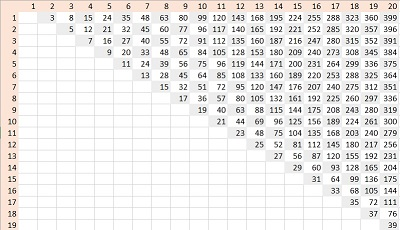# »  Solutions to puzzles in my VDARE.com monthly Diary

##November 2015

—————————

In my November diary I posed the following brain-teaser.

Representing a positive whole number as the sum of two squares is an old problem, going back at least to Diophantus. It gets three full pages in Hardy and Wright's Theory of Numbers. There is stuff all over the internet.

Representing a number as the difference of two squares is less worked over, frankly because it's easier.

So give it a try. In how many way can you express 90,000 as the difference of two squares? The numbers you're squaring should of course be positive (> zero) whole numbers.

Are there any numbers that can't be so expressed?

—————————

• Solution

90000 = a² − b²
= (a + b) × (a − b), a well-known algebraic identity;
p × q, where a = (p + q) / 2, b = (p − q) / 2

So decompose 90000 into two factors adding up to an even number (otherwise a won't be a whole number).

This can't be done with p and q both odd (because their product would be odd, and 90000 is even), or one odd one even (because their sum would be odd, which we've disallowed), so both must be even.

Now 90000 = 24*32*54, so the possibilities for (pq) are (2x, 8y), or (4x, 4y), where xy = 5625

(xy) or (yx) can be (1, 5625), (3, 1875), (5, 1125), (9, 625), (15, 375), (25, 225), (45, 125), (75, 75).

Solutions for (pq):

(2, 45000), (11250, 8), (4, 22500)
(6, 15000), (24, 3750), (12, 7500)
(10, 9000), (40, 2250), (20, 4500)
(18, 5000), (72, 1250), (36, 2500)
(30, 3000), (120, 750), (60, 1500)
(50, 1800), (200, 450), (100, 900)
(90, 1000), (360, 250), (180, 500)
(150, 600), (300, 300)

Corresponding (ab) are

(22501, 22499), (5629, 5621), (11252, 11248)
(7503, 7497), (1887, 1863), (3756, 3744)
(4505, 4495), (1145, 1105), (2260, 2240)
(2509, 2491), (661, 589), (1268, 1232)
(1515, 1485), (435, 315), (780, 720)
(925, 875), (325, 125), (500, 400)
(545, 455), (305, 55), (340, 160)
(375, 225), (300, 0)

Discarding (300, 0), since I specified positive whole numbers, the answer is twenty-two.

On the last question we can seek inspiration via experimental math. I asked Microsoft Excel to generate a² − b² for a and b in the range 1 to 20. I got this, odd results shown shaded.A glance suggests that all odd number can be so represented; and all even numbers divisible by 4.

This is easily confirmed from algebra. If n is an odd number, say 2k + 1, then n = (k + 1)² − k².

For the even case, go back to the identity a² − b² = (a + b) × (a − b). Resolving n into two factors (a + b) and (a − b), they can't both be odd (because n is even). But if one of them is even, so is the other one! Thus n is divisible by 4.

Conversely, if n is divisible by 4, say n = 4k, then n = (k + 1)² − (k − 1)², so there is always a representation.

Bottom line: if n leaves remainder 2 on division by 4, there is no way to represent n as the difference of two squares. Otherwise, excepting the special cases 1 and 4, there is.